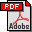Defect Theorems and Infinite Words

Jan Manuch, Defect Theorems and Infinite Words. TUCS Dissertations 41. Turku Centre for Computer Science, 2002.

Abstract:

The thesis is based on the following papers: <br>
[KMP] (TCS), [M2001] (DMTCS), [KM2002] (TCS), [CKM] (ITA) and [DM] (TCS).

<p>In the thesis we attack different problems of Combinatorics on Words.

<p>The main goal of the thesis is to look for the defect-type theorem for the bi-infinite words. In the strict sense such result does not exist: (ab)^Z=(ba)^Z. However, under the assumption that a bi-infinite word is non-periodic we are able to obtain a defect theorem for the combinatorial rank. We provide several examples showing that these assupmtions are really neccessary. We also give a complete characterization of all binary sets X allowing a double X-factorization of a bi-infinite word.

<p>In the next chapter we study necessary conditions which yield a cumulative defect effect. There are only few results in this direction: Graph Lemma and the result of [Br]. We interpreat a relation on words from X as a double X-factorization of some bi-infinite word. We ask if the fact that a non-periodic bi-infinite word possesses k disjoin X-factorizations implies that
the combinatorial rank of X is at most card(X)-k+1. We prove that the problem has an affirmative answer in the case k=2 and in the case k=3 when X is a prefix set. We also discuss connections of the case k= card(X) to Critical factorization theorem and show that the maximal number of disjoin X-factorization of a non-periodic bi-infinite word is card(X).

<p>A natural extention of word equations are language equations. We concentrate our study on the conjugacy equation XZ=ZY in the case when X and Y are binary sets. We give an example showing that even in this very restricted case we do not have a defect effect. However, we are able to
characterize all sets X, Y and Z satisfying the above conditions.

<p>In the last chapter we look at infinite words from a different perspective. We show that some open problems in the theory of computentional complexity of infinite words are equal to hard open problems of complexity theory. For example, we show that the problem if all infinite words generated by iterating dgsm's have logarithmic space complexity is equal to the problem wether the unary classes of languages P and DLOG are equivalent. Further, we separate the classes of infinite words generated by double and triple D0L TAG systems.

Files:Full publication in PDF-format

BibTeX entry:

@PHDTHESIS{phdManuch02a,
title = {Defect Theorems and Infinite Words},
author = {Manuch, Jan},
number = {41},
series = {TUCS Dissertations},
school = {Turku Centre for Computer Science},
year = {2002},
ISBN = {951-29-2288-3},
}

Belongs to TUCS Research Unit(s): FUNDIM, Fundamentals of Computing and Discrete Mathematics

Edit publication